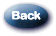The Mathematics of Computing

If you have arrived here through a search engine, and there's no menu to the left click here!

As is the case with most things involving mathematical calculations, we tend to designate numerical values by using decimal notations. As an example, each digit having one of ten values, zero through nine. This is commonly referred to as base 10 mathematics. Binary values, on the other hand, are expressed in the form of ones and zeros, and is considered to be base 2 mathematics. With base 10 mathematics, regular numbers start at 0, count to 9, and then reset the 9 to a 0 and add a 1 in the "tens" place, but with binary math you start at 0, count to 1, then reset the 1 to a 0 and add a 1 in the "twos" place. So binary counting goes like this: 0, 1, 10, 11, 100, 101, 110, 111, 1000, etc. Each digit in a binary number is a bit, a contraction of binary digit.

The number 111 is the binary equivalent of the decimal number 7. How? Just as 111 in decimal means 100 (10 to the second power) plus 10 (10 to the first power) plus 1, in binary 111 is 4 (2 to the second power) plus 2 (2 to the first power) plus 1, which is 7. As you can see, binary numbers can get pretty long. For example, the number 181 in decimal is 10110101 in binary. This can get quite cumbersome, and large numbers are often represented using 32, 64 or even more bits.

The shorthand for binary numbers is that of hexadecimal notation, which are numbers in base 16 mathematics. Each digit can take on any value from 0 to 15. Since 16 is 2 to the fourth power (24), four bits can take on a value from 0 to 15, which means 4 binary digits can be replaced by one equivalent hexadecimal digit. So 10110101 in binary can be broken into two 4-bit pairs, 1011 and 0101. These, taken individually, are 11 and 5, so 10110101 in binary is (11)5 in hexadecimal notation.

As you can see, this creates a problem as we only have 10 different symbols to represent numbers, and hexadecimal requires 16. Having to use eleven (11) in a single digit place is confusing, so to get around this, hexadecimal representation uses the letters A through F to represent 10 through 15 (0 to 9 are of course represented by 0 to 9). Therefore, instead of stating (11)5, we state that the decimal number 181 is "B5" in hexadecimal notation (or hex for short).

There are also other problems associated with decimal and hexadecimal representations, such as determining whether a number is decimal or hexadecimal merely by looking at it. As an example, if you see just the numerical "44", does that mean 44 ("44" in decimal) or 68 ("44" in hexadecimal)? To avoid this problem, two common notations are used to indicate hexadecimal numbers. The first is the suffix of a lower-case "h", and the second is the prefix of "0x". So "B5 in hexadecimal", "B5h" and "0xB5" all mean the same thing, as does the somewhat redundant "0xB5h". A set of eight bits, or two hexadecimal digits, is called a byte and "B5h" is one byte of information. Check here to see how a "byte" relates to drives.  Bytes are commonly used when discussing the amount of information a drive can hold, because in most cases one byte is one character. Each character you read on this screen is represented by one byte, and in most cases, as part of the ASCII character set.

Computer addresses are often expressed in a hexadecimal format. As an example, I/O port addresses commonly used by computers to talk to their printers is at address 378h. Note how the "h" helps you identify that this is a hex number and not the decimal number "378".Notice: Windows® 95, Windows® 98, Windows® NT, Windows® 2000 and
Microsoft® Office are registered trademarks or trademarks of the Microsoft Corporation.
All other trademarks are the property of their respective owners.